# Separable Differential Equations: An In-Depth Analysis

Solve the following separable differential equations: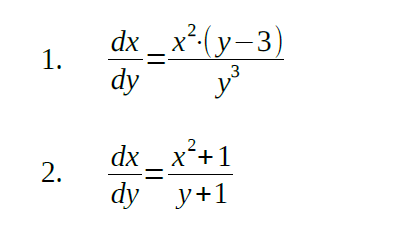Solution: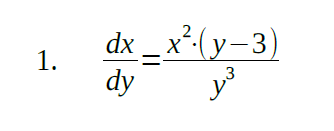Let’s rewrite the equation into the following form: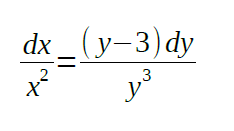The left part of the equation is a simple integral. Now, we’ll rewrite the right part: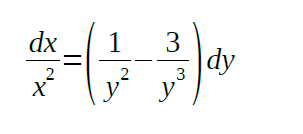Now, both parts of the equation are easy to integrate: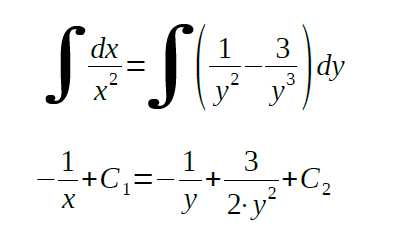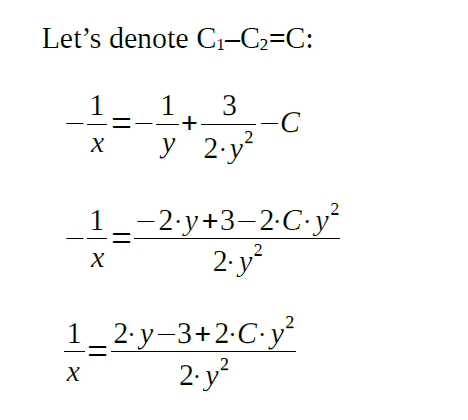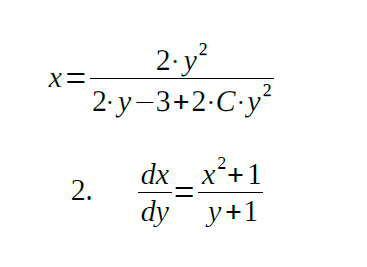Let’s reorganize the equation: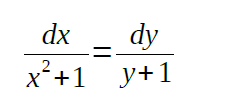Integrating both parts: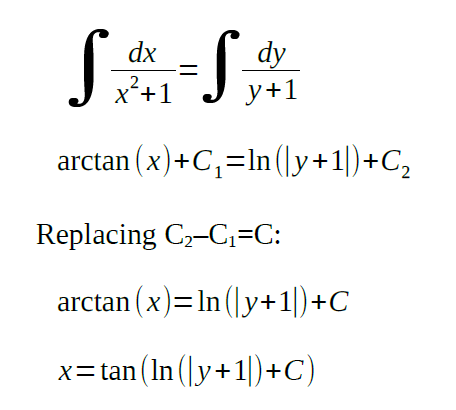This analysis of separable differential equations is provided by our experts. They also have included separable differential equations examples so that you can easily plunge into the subtleties of this complex subject. Moreover, you can use these examples in class or for another purpose.

At AssignmentShark, we provide timely help with assignments. Our experts specialize in numerous technical fields, and have been generating profound assignments and analyses for five years. Just like with this analysis containing separable differential equations problems and solutions, we can develop a comprehensive analysis in any technical field for you. If you no longer feel like dedicating your precious time to boring technical assignments, you always have our reputable service at hand!